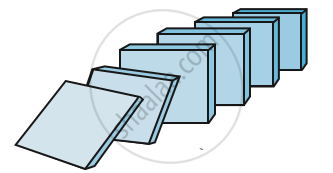# Motivation

#### description

• Motivating the Application of the Method by Looking at Natural Numbers as the Least Inductive Subset of Real Numbers

#### notes

In mathematics, we use a form of complete induction called mathematical induction. To understand the basic principles of mathematical induction, suppose a set of thin rectangular tiles are placed as shown in FigWhen the first tile is pushed in the indicated direction, all the tiles will fall. To be absolutely sure that all the tiles will fall, it is sufficient to know that
(a) The first tile falls, and
(b) In the event that any tile falls its successor necessarily falls.
This is the underlying principle of mathematical induction.
We know, the set of natural numbers N is a special ordered subset of the real numbers. In fact, N is the smallest subset of R with the following property:
A set S is said to be an inductive set if 1∈ S and  x + 1 ∈ S whenever x ∈ S. Since N is the smallest subset of R which is an inductive set, it follows that any subset of R that is an inductive set must contain N.

If you would like to contribute notes or other learning material, please submit them using the button below.